RUSSIAN JOURNAL OF EARTH SCIENCES, VOL. 12, ES4001, doi:10.2205/2012ES000516, 2012

# Plastic stress field reconstruction based on stress orientations data

2Schmidt Institute of Physics of the Earth, Russian Academy of Sciences, Moscow, Russia

### Abstract

This paper presents an investigation of the applicability of the stress trajectories concept and the stress trajectories – slip lines alternations method to geomechanical problems. We extend our approach introduced for the stress analysis of two-dimensional plastic bodies to the problem of the stress reconstruction in plastic regions of the lithosphere. The method is developed for the Cauchy boundary value problem and utilizes the data on principal directions as one of the boundary conditions. For this purpose the first order stress indicators of the World stress map (WSM) project database (release 2008) are utilized in computations. The set of considered boundary conditions is supplemented by the normal derivatives of the stress orientations. Complete formulation of the problem involves a yield condition. Although the general approach is not limited to a specific yield criterion, present calculations are performed for the Mohr-Coulomb criterion. Applications of the method include the stress reconstructions in three regions of the Earth's crust (Swiss Alps, Tibetan plateau and Eastern Anatolia). The continuous boundary conditions are derived by an averaging method applied to the discrete data in immediate vicinity of the starting boundary. Thereafter, for the chosen strength parameters of the Mohr-Coulomb theory (friction angle and cohesion), the unique grids of stress trajectories and slip lines are determined. These fields are further compared against the WSM data available inside the regions. The computations are made for different strength parameters in order to provide the best fit to the data. The results of the analysis are presented as two plane fields: the map of normalized mean stresses and the grid of corresponding trajectories of principal directions. The normalization parameter is unknown (it represents an initial value of the mean stress in a single node of the boundary), which is a consequence of non-uniqueness of the stress reconstruction problem based on the data on stress orientations alone. The reconstructed stress orientations are compared with the observations from the WSM database.

### 1. Introduction

Identification of stress fields inside tectonic plates is an essential part of any global relative-plate-motion model [Gordon, 2000; Sutherland, 2008] as it serves as an important indicator of plate-driving forces. Numerous authors [Becker et al., 2005; DeMets et al., 2005; Flesch et al., 2007; Kreemer et al., 2003; Lund and Townend, 2007; Mukhamediev et al., 2005; Steinberger et al., 2001] discuss the importance of studying lithospheric stresses and their impact on geodynamics of the Earth crust. This study is aimed at the identification of tectonic stresses in some regions of the globe that could be considered as plastic. First order stress indicators of the World Stress Map (WSM) project database [Heidbach et al., 2008] are utilized to evaluate the magnitudes of intraplate stresses. The numerical method is based on the stress trajectory concept and presents further development of the approaches reported by Galybin and Mukhamediev  for elastic solids and by Haderka and Galybin  for plastic media.

Methods widely accepted for stress modeling are based on finite element analyses in view of gravitational potential energy (GPE) predictions [Bird, 1989, 1999; England and Molnar, 1997; Humphreys and Coblentz, 2007]. The main effort, as identified by Sonder and Jones , is invested into identifying the forces driving and resisting the inter- and intra-plate deformations. Different estimates of the boundary forces [Coblentz et al., 1995], forces arising from lateral density variations [Andeweg et al., 1999; Gölke and Coblentz, 1996] and their combinations [Vergnolle et al., 2007] are chosen such that the model predictions fit the observed stress indicators. Justifications of the methods in view of WSM data were shown in [Chang and Lee, 2010; Cloetingh and Wortel, 1985; Coblentz and Sandiford, 1994; Zoback et al., 2002]. Alternatively, velocities determined by the Global Positioning System (GPS) at different sites could be utilized [DeMets et al., 1990; England and Molnar, 2005; Flesch et al., 2001; McClusky et al., 2003].

However, model predictions resulting from various estimates of driving forces lead to inconsistent and contradictory results [Sonder and Jones, 1999]. The common sign described in Gölke and Coblentz , is twofold, (1)  the invariant nature of the stress orientations, and (2) the great dependence of the stress magnitudes, to the boundary conditions used. Moreover, often the methods are based on elastic assumption regarding the lithosphere, whereas Vergnolle et al.  argue that the differences between model predictions and stress indicators may also arise from non-elastic rheology. Pauselli et al.  report, that the composition, structure, temperature and deformation conditions all affect the rheology.

In contrast to FEM based GPE prediction methods, we suggest using the WSM stress indicators as boundary conditions in the direct formulations of boundary value problems (BVP) and hence overcoming the necessity for estimation of boundary conditions crucial in inverse problems. We propose to employ a plastic model with friction to describe the state of failure equilibrium of intraplate continental crust. In this case, the governing equations consist of the differential equations of equilibrium (DEE) and a criterion that imposes certain algebraic relationships on the stress components. The assumption of plastic rheology has been accepted in [Hieronymus et al., 2008; Molnar and Tapponier, 1977; Stein et al., 1992; Zoback et al., 2007]. None of these studies however made direct use of the WSM data for the formulation of BVP. It is explained by the fact that the classical formulations assume specification of boundary stresses while the majority of data are given on the stress orientations, therefore restricting the straightforward application of the conventional techniques. In the approach proposed by Haderka and Galybin  the orientations of principal stresses are considered as one of the possible boundary conditions for solving the BVP of plane plasticity. This opened a possibility to model non-elastic stress fields without knowing reliable information on the boundary stress magnitudes.

Fundamentals of the non-elastic stress modeling are developed in works of Hill  and Sokolovskii . In particular, it is shown that for two dimensional problems (in which the conditions of limiting equilibrium apply) the governing equations are presented by the system of partial differential equations (PDE) of hyperbolic type. Two distinct families of the characteristics of this system coincide with the slip lines, which are also observed by experiments. For hyperbolic systems of PDE, several BVP can be formulated, the key one being the Cauchy BVP. In this case the problem consists of solving a system of two PDE (that is obtained by substitution a yield criterion into the DDE) with certain conditions prescribed along a starting boundary (which is nowhere coincident with a characteristic of the system). When only statically determined bodies are considered the solution can be built independently of the kinematic equations. For briefness the conventional methods are further abbreviated by SL (slip lines). The solution of the Cauchy BVP by means of the SL approach is restricted to an area referred to as the characteristic triangle (or the domain of dependence). This area is bounded by the starting boundary and two characteristics of different families emanating from the ends of the boundary. It has to be emphasized that it is not straightforward to determine the solution beyond the boundaries of the characteristic triangle. Other, additional assumptions have to be taken into account as e.g. by Cox  or more recently by Martin .

In contrast to the SL approach we propose to build a solution to the problem by using the trajectories of principal stress. The stress trajectories, according to Frocht , are defined as curves the tangents to which at every point coincide with the directions of principal stresses. Because there exist two principal stresses in plane problems, there will be two distinct and orthogonal families of trajectories; one corresponding to the major and another to the minor principal stress. Similarly to the SL approach, the solution of the BVP along stress trajectories leads to a network built by the two orthogonal families. However, this network does not coincide with the characteristic grid. In other words, there exist regions of the reconstructed stress trajectories grids located outside of the domain of dependence. The implications of this fact are discussed in great detail in Haderka and Galybin . This approach is abbreviated as ST (stress trajectories) further on.

We further use both approaches in turn and introduce the ST-SL alternations method (for detail see [Haderka and Galybin, 2011]). It is shown how slip lines and stress trajectories built by the respective approaches are utilized for further extension of the stress field. Numerical tests revealed that the accuracy of this method is comparable to that observed in the conventional SL approach, which is explained by the similar finite difference techniques used.

Applications of the developed approaches are presented for the problems of stress field identification in plastic regions of the lithosphere. Following Regenauer-Lieb  it is assumed that the rheology of the lithosphere satisfies the Mohr-Coulomb theory of limiting equilibrium and hence can be modelled as a plastic medium with friction and cohesion. These parameters can be found by fitting the predicted stress orientations to the WSM data. The results of the analysis and the comparisons with the available data are shown in section 4.

### 2. Background

#### 2.1. SL Approach in Plastic Media

The DEE for a plane problem can be written in terms of the mean stress $P$ and the maximum shear stress $\tau$ in the following form:

\begin{eqnarray*} \partial_x P + \cos(2\theta)\partial_x \tau + \sin (2\theta)\partial_y \tau - 2 \tau \sin (2\theta)\partial_x \theta + 2 \tau \cos (2\theta)\partial_y \theta = 0, \\ \end{eqnarray*}

\begin{equation} \tag*{(1)}\label{1} \partial_x P + \sin(2\theta)\partial_x \tau - \cos (2\theta)\partial_y \tau + 2 \tau \cos (2\theta)\partial_x \theta + 2 \tau \sin (2\theta)\partial_y \theta = 0. \end{equation}

Here, symbols $\partial_x$, $\partial_y$ stand for differential operators. The stress functions $P, \tau$ are functions of the Cartesian coordinates $x,\: y$. They represent the sum and the difference of the principal stresses $\sigma_1$, $\sigma_2$, respectively:

\begin{equation} \tag*{(2)}\label{2} P= \frac{\sigma_1 + \sigma_2}{2}, \;\; \tau = \frac{\sigma_1 - \sigma_2}{2}, \;\; (\tau \geq 0, \; \sigma_2 \leq \sigma_1) \end{equation}

Angle $\theta$ denote the orientation of the major principal stress $\sigma_1$ measured counter-clockwise with respect to the positive direction of the $x$-axis (it is accepted that compression is negative).

In problems of plane plasticity a yield criterion has to be specified additionally to equation (1). In the works by Hill  and Sokolovskii , where the solution of this system for plastic media is developed, the Mohr-Coulomb yield criterion is utilized. It can be presented in the form:

\begin{equation} \tag*{(3)}\label{3} \tau = C \cos\mu - P \sin \mu, \;\; \tau \geq 0 \end{equation}

where the maximum shear stress is expressed as a linear function of the mean stress via the angle of internal friction, $\mu$, and cohesion, $C$. It should be noted that yielding criterion (3) is valid if the maximum shear stress in (3) does not vanish and the normal stresses on the slip lines are compressive (negative).

Integration of (1) in view of (3) and the boundary conditions leads to four characteristic equations; two of them for identification of the slip lines:

\begin{equation} \tag*{(4)}\label{4} dy = dx \tan (\theta \pm \varepsilon) \end{equation}

and two for the relationships valid along the slip lines:

\begin{equation} \tag*{(5)}\label{5} dP^* \pm 2 P^* \tan\mu d\theta =0 \end{equation}

Equations (5) define the relationships between the mean stress and the principal directions valid along the slip lines ($P^*$ represents the mean equivalent stress, $P^* = P - C \cot \mu$). Equations (4) define two families of isogonal lines inclined to the direction of the major principal stress $\sigma_1$ at the angles:

\begin{equation} \tag*{(6)}\label{6} \pm \varepsilon = \frac{\pi}{4} + \frac{\mu}{2} \end{equation}

From the latter relationship it is evident that this inclination depends on the angle of internal friction.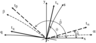Figure 1
Solution of systems (4) and (5) is performed numerically by employing the finite difference method (see [Sokolovskii, 1965] for detail). Equations (4) allow for the identification of nodal points represented as intersections of tangents to the slip lines (emanating from boundary nodal points, see Figure 1). Reconstructed slip lines form a zone of limiting equilibrium presented by a grid where two characteristics of different families intersect at the angle $2 \varepsilon$. The resulting stress field is found in an area that has the form of a curvilinear triangle. As mentioned previously, this area is referred to as the characteristic triangle or the domain of dependence.

For completeness it has to be mentioned that if the angle $\theta$ in (4) is:

\begin{equation} \tag*{(7)}\label{7} \theta \pm \varepsilon = \frac{m}{2} \pi, \;\; m= 0,\:1,\:2, \ldots \end{equation}

then the SL approach fails as the nodal points can not be identified. Since (7) is valid everywhere along the slip lines, none of these lines can be used as a stand-alone starting boundary.

#### 2.2. Integration of the DEE Along Stress Trajectories

The present approach is based on utilizing the stress trajectories instead of the slip lines in solving the BVP introduced in the previous section. The relationship between the orthogonal families of stress trajectories (specified by the tangents $t_s$, $t_q$) and the slip lines (specified by the tangents $t_{\alpha}$, $t_{\beta}$), are given as:

\begin{equation} \tag*{(8)}\label{8} \hat{\alpha} = \theta-\varepsilon, \;\; \hat{\beta} = \theta+\varepsilon \end{equation}

where $\hat{\alpha}, \hat{\beta}$ represent the tangent angles between the slip lines $\alpha$ and $\beta$ and the $x$-axis, respectively (see also Figure 1). The system in (1) can be rewritten along the stress trajectories, hence representing the Lamé-Maxwell form of the DEE (e.g. in [Kuske and Robertson, 1974]), which can be written as:

\begin{equation} \tag*{(9)}\label{9} a\partial_s P + \partial_q \theta =0, \;\; b\partial_q P + \partial_s \theta =0 \end{equation}

The arc lengths $s, \:q$ are measured along the stress trajectories of the first and second family, respectively. The coefficients and are functions of spatial coordinates; for the Mohr-Coulomb yield criterion they have the form:

\begin{equation} \tag*{(10)}\label{10} a = \frac{1}{2} \frac{1- \sin \mu}{C \cos \mu - P \sin \mu}, \;\; b = \frac{1}{2} \frac{1+ \sin \mu}{C \cos \mu - P \sin \mu} \end{equation}

Equations (9) in view of (10) form a closed system and, together with the boundary conditions, constitute the BVP for plastic media.

A method for solution of (9) along the stress trajectories for a general, explicit yield criterion has been developed by Haderka and Galybin . Here we focus on the Mohr-Coulomb criterion (3) and the boundary conditions specified in terms of orientations of the principal stress and their normal derivatives $(\theta, \, \partial_n \theta$).

Similarly to SL, the solution is performed numerically by the finite difference approach and it is decoupled into two steps for the determination of the stress trajectories and the relationships along them. Based on the definition of the stress trajectories, the nodal points are sought as the points of intersections of two different, orthogonal families which emanate from neighboring nodes on the boundary. The curvilinear families of trajectories are approximated by polygons, hence the used piecewise linear approximation introduces some errors in the identification of the intersection points of trajectories. However, these errors are not significant and they are of the same order as in the SL approach where the same approximation is accepted (see (4)). The mean stresses and the orientations are calculated at the new nodes by continuation of their boundary values by using expansions into Taylor's series along the stress trajectories (derivatives of the order greater than one are neglected). The introduced directional derivatives along the trajectories ($\partial_s$ and $\partial_q$) are then transformed into the tangential and normal derivatives with respect to the contour ($\partial_t$ and $\partial_n$), respectively). Once the derivatives of the orientations are known ($\partial_t \theta$ can be retrieved from the information along the starting boundary and $\partial_n \theta$ is specified in boundary conditions) the derivatives of the stresses are determined from (9). Hence, the determined derivational terms satisfy the DEE and are consequently used to recalculate the sought mean stresses and the orientations at the new nodal points. However, it has to be noted that the mean stress $P$ can be determined only in a normalized form, thus its initial value remains unknown. With a sufficiently dense stress trajectories network the set of values ($P_{j,k}$ and $\theta_{j,k}$) at every point $z_{j,k}$ presents an approximate solution to the BVP. An overview of the equations obtained by the described numerical integration of the BVP and the inherent limitations are presented in Appendix A.

#### 2.3. Comparison of SL and ST Approaches for Linearly Varying Boundary Conditions

In order to illustrate that both approaches produce close results we conduct numerical tests for the Mohr-Coulomb criterion. Boundary conditions are given in terms of orientations and their normal derivatives. It is assumed that they both have linear distributions along the starting boundary, i.e. $\theta = ax +b$ and $\partial_n \theta = cx +d$ on a part of the $x$-axis. Following the classical approaches it is also assumed that all the variables having the dimension of stresses are normalized with respect to cohesion, $C$.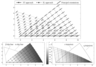Figure 2
Typical solutions to systems (9), (3) and (1), (3) obtained by using the ST and SL approaches, respectively, are shown in Figure 2. For visual comparison, the results are plotted for the stress trajectories grid (full lines with circles) and the slip lines grid (dashed lines with dots). Orientations of the major principal stress obtained by the different approaches are shown by short line segments (Figure 2, top). Additionally, the reconstructed fields of the mean stresses are presented in the bottom pictures in Figure 2.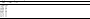Table 1
From the top picture in Figure 2 it is evident that the results of the different approaches are obtained at different nodes. Therefore a numerical procedure is developed in order to compare these results. It involves linear interpolation of the values obtained by the SL approach at the nodal points found in the ST approach. Triangular elements are built for this purpose, such that the nodes of the SL approach present their vertices. Linear interpolation is performed to find the stress orientations at the nodes of the ST approach, lying inside the triangular elements. The results of comparisons are summarized in Table 1. Columns 2 and 3 of the table show the maximum observed differences obtained by the two approaches for orientations ($\Delta \theta$) and stresses ($\Delta P$), respectively, depending on the increasing number of boundary nodal points. It has been found that the maximum differences $\Delta \theta$ and $\Delta P$ decrease with the denser discretisation (number of nodes $n$ increases) of the boundary.

The results obtained by the two approaches are in good agreement, however, the deviation of the results may increase (depending on the boundary conditions) when the nodes in the ST approach cross the boundaries of the domain of dependence (specified by the slip lines). These observations suggest that, while the results of the ST approach are unique, the identified stress field might comprise regions (lying outside of the domain of dependence of the boundary segment) where the real stress field is no longer dependent on the boundary conditions along the starting boundary. Therefore, the solution in these regions, obtained by the ST approach, can be considered as an extrapolation of the real solution (see [Haderka and Galybin, 2011] for more details).

### 3. Reconstruction Based on Alternations of SL and ST Approaches

The method of alternations is developed for the starting boundaries along which relationship (7) are valid in the case of the slip lines or (A.8) for the case of the stress trajectories. It is shown that the mean stresses and the stress orientations identified along these lines can be utilized for further extension of the stress field (beyond the boundaries of the reconstructed triangles). For this purpose it is assumed that the whole domain is in state of limiting equilibrium and that the boundary conditions allow for such extension. In the following examples boundary conditions along the starting boundary are specified according to Figure 2.

#### 3.1. Stress Trajectory as a Starting Boundary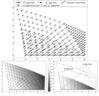Figure 3
Let us assume that the stress trajectories grid, shown in Figure 2, and, in particular, the legs of the characteristic triangle, are known. While the ST approach can not utilize this information because of the limitation in (A.8), there are no restrictions in the SL approach to use these curves as starting boundaries to perform computations. In Figure 3, (top) two additional characteristic grids (to those in Figure 2) are shown which emanate from the $s$-trajectory and from the $q$-trajectory (dash-dotted lines with squares).

Maps of the mean stress in the extended region and inside the characteristic triangle built from the starting boundary are presented in Figure 3 (left) and (right), respectively. The contours of triangles obtained by the different methods are emphasized with full thick lines. The results obtained for different starting boundaries are tested for accuracy as described in the following sub- section.

#### 3.2. Slip Line as a Starting Boundary

In the case when the starting boundary is an existing slip line, both numerical procedures, developed in previous sections for the ST and SL approaches, fail to give reliable results. However, the ST approach can be modified following the idea mentioned in Haderka and Galybin .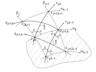Figure 4
Let us consider a field of slip lines obtained from calculations by the SL approach. One possible configuration is presented in Figure 4, where only a part of a slip field is shown (represented by slip lines $\alpha_{k-1}, \, \alpha_{k},\, \alpha_{k+1}$ and $\beta_j, \, \beta_{j+1}$). Taking into account the known relationships between the slip lines and the stress trajectories, one can find the nodal points $z_{j, k}$ as intersections of the former with the latter.

Slip lines between the layers $k$ and $k-1$, are given by the nodes $z_{j, k-1}$, $z_{j+1, k-1}$ and $z_{j, k}$, $z_{j+1, k}$ available from the previous calculations (they belong to the characteristic triangle). The nodes $z_{j, k+1}$, $z_{j+1, k+1}$ and the slip line $\alpha_{k+1}$ which passes through them are unknown. Because of the expression in (8) the position of the stress trajectories $s_{j,k}$, $q_{j,k}$ is known at these nodes. Therefore, the nodal points $z_{j, k+1}$, $z_{j+1, k+1}$ can be found as the intersections of $s_{j,k},\: \beta_{j+1}$ and $q_{j+1,k},\: \beta_{j}$, respectively. After identification of $z_{j, k+1}$, $z_{j+1, k+1}$, the mean stresses and the orientations are sought at these points by employing a finite difference form of DEE (9). Details of the numerical analysis together with the corresponding relationships are presented in Appendix B.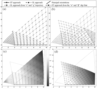Figure 5
An application of this method for the Mohr-Coulomb criterion is presented in Figure 5. The slip lines grid in Figure 2 (top) is considered and the legs of the characteristic triangle are taken as the starting boundaries. The solution proceeds layer by layer until the nodal points of the ST approach (dashed lines with crosses), and the stresses and orientations at them, are found.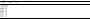Table 2
In Figure 5b we also provide an overview of all the grids obtained by the approaches at hand. It can be observed that the stress trajectories built for different starting boundaries are in perfect correlation. The same applies for the slip lines. A numerical comparison of the $P$- and $\theta$-fields at the nodal points of the different approaches has also been performed and the maximum absolute errors are given in Table 2.

The results are in good agreement in the areas where the identified stress trajectories and the slip lines grids overlap.

### 4. Modeling of the Stress Fields in Regions of the Earth's Crust

In this section the developed approaches are applied to the problem of stress reconstruction in some areas of the Earth's crust. Three particular regions are considered and investigated in view of the WSM data. As there is no information available regarding the stress magnitudes, the boundary conditions are posed in terms of $\theta$ (WSM database release 2008 is considered, [Heidbach et al., 2008]) and $\partial_n \theta$. Moreover, only the data of quality A-C (see details of data classification in [Zoback and Zoback, 1991]) are taken into account.

Computational areas chosen for the analysis are the Alps region, a region in Tibet (both parts of the Eurasian plate) and a region in Eastern Turkey (a part of the Anatolian and Arabian plate). Lithospheric body forces, from which the stresses arise, are a consequence of high topography of these regions, as each of these areas belongs to a mountain massif. Therefore, the methods in plasticity can be considered as more adequate. Moreover, following the research of Ghosh et al. , these areas represent the zones of strike-slip type of faulting which are dominated by plastic deformation [Fossen, 2010]. Characteristic sizes of all these areas are significantly less than the radius of the Earth, therefore they can be considered in plane assumption.

Application of the method of alternation is performed for straight starting boundaries. This simplification is not vital but rather used because of the nature of the data (which are scattered in the whole region). An averaging method is introduced to transform the discrete data into continuous boundary conditions. For this purpose the moving window method is used. Thus, an elongated rectangle is introduced at the vicinity of the starting line and all WSM data falling within this rectangle are selected. Consequently, the mean of the orientations inside the area is associated with the boundary condition $\theta$ and the standard deviation is associated with the normal derivative of the principal orientations, $\partial_n \theta$.

The numerical calculation begins with the identification of the stresses along the starting boundary from (A.3) and (A.4). Here, the strength parameter $C$ is chosen to be equal to unity during the whole analysis whereas $\mu$ varies between $5\mbox{°}$ and $40\mbox{°}$ in order to achieve the best correlation of the results. The free parameter in (A.3); namely the mean stress $P_{j, k}$, can be chosen arbitrarily (in the calculations the value is set to unity).

Solution proceeds in accordance with relationships (A.2)–(A.5) till the stress trajectory grid is built. Using the idea of alternations, the identified legs of the stress trajectories triangle are taken as starting boundaries for the SL approach. Next, using the slip lines reconstructed by the SL approach, the stress field is extended by the ST approach as described in sub-section 3.2 (similarly to Figure 5). The results of the calculations for different regions are presented in the following sub-sections.

#### 4.1. Alps region – 6° E–10° E and 46° N–49° N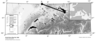Figure 6
Based on observations available for the western European stress province, the stress orientations are characterized by almost homogeneous NW-SE inclinations [Ahorner, 1975; Müller et al., 1997]. This feature of the West European stress field is however locally disturbed by the Alpine geologic structure [Grünthal and Stromeyer, 1992; Müller et al., 1992]. We focus on the Swiss Alps region with the computation domain bounded by the lakes Konstanz and Geneva on the north-east and north-west, respectively and lake Maggiore on the south (see Figure 6).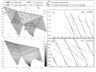Figure 7
The solution obtained for this region by the ST-SL alternation method is shown in Figure 7. Boundary conditions identified along the starting boundary (specified along the full thick line in Figure 6) are $\theta_{j,0}=109\mbox{°}$ and $\partial_n \theta_{j,0}=0.46$. The results of the analysis are shown for the friction angle of $\mu = 15\mbox{°}$.

The stress trajectories grid, presented by dots in Figure 7 (top, left), is built from the starting boundary. Nodes of the slip lines grid are presented by circles. It can be noted that the starting boundaries of the SL approach coincide with the stress trajectories. Finally, information along the slip lines is used to extend the solution area once more. These grids are presented by the nodes in the form of small triangles. The map of the mean stresses is shown in Figure 7 (bottom, left). The pictures on the right (Figure 7) present two inserts that show the predicted and observed stress orientation data used for the analysis of the accuracy in the stress field reconstruction.

#### 4.2. Tibet Region – 75° E–95° E and 24° N–44° N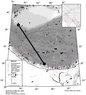Figure 8
The extensive Himalayan-Tibet dataset of the WSM project (see Figure 8) shows that the region of interest is dominated by a N–S oriented stress field in the centre of Tibetan plateau [Chamlagain and Hayashi, 2007] with a slight eastern trend south of the Tarim basin. These observations agree well with the GPE-prediction based models of Ghosh et al.  and the quasi-rigid block-model based on GPS data reported in Thatcher . We focus on the region located between the Tarim basin on the north and the Himalayas on the south.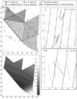Figure 9
The reconstructed grids of slip lines and stress trajectories and the identified stress distribution for this region are shown in Figure 9. Here, the same style as in the preceding sub-section is adopted for marking the results obtained by different methods. The results are shown for $\mu = 25\mbox{°}$, the boundary conditions found from the data analysis are $\theta_{j,0}= 79.89\mbox{°}$ and $\partial_n \theta_{j,0} = 0.08$.

#### 4.3. Eastern Anatolia – 33° E–51° E and 31° N–45° N

The Eastern Anatolia region represents an example of a continental collision zone [Keskin, 2007]. The analysed area lies in eastern part of the Anatolian plate and extends to the Arabian plate. The former, being surrounded by the Eurasian, African and Arabian plate, has very active tectonics. Stress regime in the study area has a strike-slip character. Results by Yilmaz et al.  predict ENE-trending of stress in Eastern Anatolia, whereas, the Five Domain model by Dwivedi and Hayashi  gives the principal stresses trending NE to E.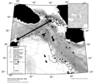Figure 10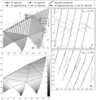Figure 11
The starting boundary ranges from the Anatolian-Arabic-African triple junction up to the eastern termination of the Northeast Anatolian Fault Zone and it runs alongside the Eastern Anatolian Fault Zone (Figure 10). For the third investigated region the analysis of the data gave the following conditions along the starting line, $\theta_{j,0}= 82.33\mbox{°}$ and $\partial_n \theta_{j,0} = 0.1$. The results are shown in Figure 11 for the friction angle of 27°. The marker style is adopted from the sub-section 4.1.

As evident from the figures in this example, in some regions the results correlate to a good degree of accuracy whereas in others the accuracy of the predicted orientations is fair. However, it follows directly from the nature of the observed orientations that a good correlation cannot be achieved in this area because of the randomness present in the data. One way to improve the certainty of the results is to use the data of the highest quality (WSM rank A) in this region. However, it has to be noted, that the inaccuracies can also stem from the choice of the constitutive law (rheology), adoption of the 2D model or other, including geological, effects.

### 5. Concluding Remarks

Applicability of the ST-SL alternation method, developed for the limiting equilibrium analysis of two-dimensional plastic bodies, has been studied to address the geomechanical problem of stress reconstruction in the Earth's crust. Namely, identification of stress fields in mountain regions of the crust has been of the main concern. Discrete data on stress orientations taken from the WSM database have been utilized for numerical analysis, the stress magnitudes remained unknown. The data have been treated by a specially developed procedure to obtain continuous functions of the orientations of the principal stress and their normal derivatives along the starting boundary. As a result, the proposed numerical procedure allowed for the unique identification of the stress trajectories grid (or the slip lines grid). The mean stresses are found in the normalized form such that the normalization parameter remains unknown, which emphasizes the non-uniqueness of the stress reconstruction problem based on stress orientations alone.

The numerical scheme has been developed for the Mohr-Coulomb criterion which is widely accepted for modeling of stress fields in plastic regions of the lithosphere. The criterion contains two parameters controlling the stress fields; the angle of internal friction and cohesion. The latter has been used for normalization of stresses, while the former can be estimated from the best fit of the predicted stress orientations and the external data, i.e. for data that have not been involved in the identification of the boundary conditions by the moving window method. It should be noted that the Mohr-Coulomb yield condition does not limit the ST approach and the ST-SL alternations method. It has been proved in previous studies that some other criteria are also admissible.

The method has been applied to reconstruct plastic stress fields in three different regions of the Earth's crust. In particular, the best-fit model of the Swiss Alps region predicts the NNW stress orientation with a NW trending near Lake Maggiore. For the vast part of the Tibetan plateau, the maximum compressive stresses are oriented in North direction with the exception of the south-eastern part where a NNW trend has been observed. Stress orientations in the eastern Anatolia and Arabian plate show NNE to NE directions. In general, in all the regions analysed, the numerical modeling satisfactorily predicts the data on the WSM stress indicators (calculated relative errors are within the expected errors inherent in the data). However, the predictions are less accurate for the north-eastern Anatolian region which can be explained by the high level of WSM data scattering.

The analysis performed on the basis of plastic rheology has shown satisfactory agreement between the predicted and observed stress orientations in some mountain regions of the lithosphere. This justifies the existing opinion [Fossen, 2010] that plastic deformations are dominant in geodynamics of the regions of strike-slip type of faulting. In comparison with the conventional methods the applied numerical procedure leads to mechanically justified solutions within significantly large areas (in comparison with the conventional methods, SL formulations, of two dimensional plasticity). It is important that the method allows for the reconstruction of the fields of maximum shear stresses and mean stresses, which cannot be obtained by any pure interpolation or statistical methods.

#### Acknowledgments

The work is partly supported by the RFBR Grant 11-05-00970.

### Appendix A: Numerical Solution by the ST Approach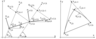Figure 12
Nodal points of the solution are sought as the intersections of two different families $s_{j,k}$ and $q_{j+1,k}$ (see Figure 12, left for notations) which emanate from two neighboring nodal points on the boundary. The unknown node $z_{j,k+1}$ near the vicinity of the boundary is sought as:

\begin{eqnarray*} z_{j, k+1}= z_{j,k} + \delta^{(s)}_{j,k} e^{i \theta_{j,k}} \end{eqnarray*}

\begin{equation} \tag*{(A.1)} z_{j, k+1}= z_{j+1,k} + i \delta^{(q)}_{j+1, k} e^{i \theta_{j+1,k}} \end{equation}

The fact that both relationships in (A.1) should specify the same nodal point allows for the determination of the unknown distances $\delta_{j,k}^{(s)}$, $\delta_{j+1,k}^{(q)}$ as follows:

\begin{eqnarray*} \left( \begin{array}{c} \delta_{j,k}^{(s)} \\ \\ \delta_{j+1,k}^{(q)} \end{array} \right) = \frac{1}{\cos(\theta_{j,k} - \theta_{j+1,k})} \times \end{eqnarray*}

\begin{equation} \tag*{(A.2)} \left( \begin{array}{cc} \cos \theta_{j+1,k} &\sin \theta_{j+1,k} \\ \\ \sin \theta_{j,k} & - \cos\theta_{j,k} \end{array} \right) \left( \begin{array}{c} {\rm Re} (z_{j+1, k} - z_{j, k}) \\ \\ {\rm Im} (z_{j+1, k} - z_{j, k}) \end{array} \right) \end{equation}

The accuracy of the utilized piecewise linear approximation is illustrated in Figure 12 (right). The mean stresses and the principal directions at the new nodal points $z_{j, k+1}$ are calculated by means of the Taylor's expansion as:

\begin{equation} \tag*{(A.3)} \left( \begin{array}{c} P_{j,k+1} \\ \\ \theta_{j,k+1} \end{array} \right) = \left( \begin{array}{c} P_{j,k} \\ \\ \theta_{j,k} \end{array} \right) + \delta_{j,k}^{(s)} \left( \begin{array}{c} \partial_s P_{j,k} \\ \\ \partial_s \theta_{j,k} \end{array} \right) \end{equation}

Derivatives of the order higher than one are neglected. Derivational terms on the right-hand side of (A.3) are unknown and are yet to be determined. Based on the boundary conditions, posed in terms of orientations $\theta$ and their normal derivatives $\partial_n\theta$, the numerical procedure utilizes the transformation formulas between the directional derivatives along the stress trajectories $(s,q)$ and the tangential and normal directions to a contour ($t,n$). DEE (9) in view of this transformation (DEE in terms of normal and tangential derivatives) can be written in the form:

\begin{eqnarray*} \left( \begin{array}{c} \partial_t P_{j,k} \\ \\ \partial_n P_{j,k} \end{array} \right) = \left( \begin{array}{cc} a_{j,k}\cos \xi_{j,k} & a_{j,k} \sin \xi_{j,k} \\ \\ -b_{j,k} \sin \xi_{j,k} & b_{j,k} \cos\xi_{j,k} \end{array} \right)^{-1}\times \end{eqnarray*}

\begin{equation} \tag*{(A.4)} \left( \begin{array}{cc} + \sin \xi_{j,k} & - \cos\xi_{j,k} \\ \\ - \cos\xi_{j,k} & - \sin \xi_{j,k} \end{array} \right) \left( \begin{array}{c} \partial_t \theta_{j,k} \\ \\ \partial_n \theta_{j,k} \end{array} \right) \end{equation}

or equivalently:

\begin{eqnarray*} \left( \begin{array}{c} \partial_n P_{j,k} \\ \\ \partial_n \theta_{j,k} \end{array} \right) = \left( \begin{array}{cc} \sin \xi_{j,k} & - \cos \xi_{j,k} \\ \\ -b_{j,k} \cos \xi_{j,k} & a_{j,k} \sin \xi_{j,k} \end{array} \right)^{-1}\times \end{eqnarray*}

\begin{equation} \tag*{(A.5)} \left( \begin{array}{cc} - a_{j,k} \cos \xi_{j,k} & \sin \xi_{j,k} \\ \\ b_{j,k} \sin \xi_{j,k}& - \cos\xi_{j,k} \end{array} \right) \left( \begin{array}{c} \partial_t P_{j,k} \\ \\ \partial_t P_{j,k} \end{array} \right) \end{equation}

It should be noted that the tangential derivatives in the equations above can be expressed via the values of $\theta$ and $P$ on the $k$-th layer by the forward finite differences:

\begin{equation} \tag*{(A.6)} \left( \begin{array}{c} \partial_t P_{j,k} \\ \\ \partial_t \theta_{j,k} \end{array} \right) = \frac{1}{|z_{j+1,k} - z_{j,k}|} \left( \begin{array}{c} P_{j+1,k} - P_{j,k} \\ \\ \theta_{j+1,k} - \theta_{j,k} \end{array} \right) \end{equation}

Notations $a_{j,k}, \; b_{j,k}$ refer to the values at the node $z_{j,k}$ of the corresponding coefficients introduced in (10). It can be readily observed that by substitution of the second equation in (A.6) into (A.4) the tangential and normal derivatives of $P$ can be found directly. These derivational terms (along the tangent and the normal) specify the directional derivatives in the Taylor's expansion (A.3). As the result, the unknown stress field $P$ is found in the normalized form such that the initial value of $P_{j,k}$ remains unknown.

Since (A.4) and the boundary conditions now allow for recalculation of the stresses from (A.3) along the starting boundary the solution further continues with equations (A.5) and (A.6) (where, the unknown normal derivatives are sought as functions of the known values along the boundary).

One obvious limitation of the method is the existence of the inverse matrix that becomes singular if its determinant vanishes, which yields:

\begin{equation} \tag*{(A.7)} a_{j,k} b_{j,k}=0 \Leftrightarrow\cos \mu =0 \end{equation}

in (A.4) or:

\begin{equation} \tag*{(A.8)} a_{j,k} - b_{j,k} - (a_{j,k} + b_{j,k}) \cos 2 \xi_{j,k} =0 \end{equation}

in (A.5). Note, that the restriction in (A.7) implies non-physical values for the friction angle that usually varies in the range $(0, \pi/4)$.

### Appendix B: Numerical Analysis – Starting From a Slip Line

The finite difference form of equation (9) written with respect to the point $z_{j,k}$ and along trajectories $s_{j,k}$, $q_{j+1,k-1}$, yields:

\begin{eqnarray*} a_{j,k} \frac{P_{j+1,k+1} - P_{j,k}}{\Delta s_{j,k}} + \frac{\theta_{j,k} - \theta_{j+1,k-1}}{\Delta q_{j+1,k-1}} =0, \end{eqnarray*}

\begin{equation} \tag*{(B.1)} b_{j,k} \frac{P_{j,k} - P_{j+1,k-1}}{\Delta q_{j+1,k-1}} + \frac{\theta_{j+1,k+1} - \theta_{j,k}}{\Delta s_{j,k}} =0. \end{equation}

Here, $\Delta s_{j,k} = | z_{j+1, k+1} -z_{j,k}|$ and $\Delta q_{j+1,k-1} = | z_{j, k} -z_{j+1,k-1}|$ determine the distances between the given nodes (see Figure 4). Similarly this scheme can be applied for the trajectories $s_{j,k-1}, \; q_{j+1, k}$ at the point $z_{j+1, k}$ which gives:

\begin{eqnarray*} a_{j+1,k} \frac{P_{j+1,k} - P_{j,k-1}}{\Delta s_{j,k - 1}} + \frac{\theta_{j,k+1} - \theta_{j+1,k}}{\Delta q_{j+1,k}} =0 \end{eqnarray*}

\begin{equation} \tag*{(B.2)} b_{j+1,k} \frac{P_{j,k+1} - P_{j+1,k}}{\Delta q_{j+1,k}} + \frac{\theta_{j+1,k} - \theta_{j,k-1}}{\Delta s_{j,k-1}} =0 \end{equation}

Because of the piecewise linear approximation adopted for the ST approach, some errors are introduced. The averages of the solutions in (B.1) and (B.2) at the node $z_{j+1, k+1}$ have been used to improve accuracy.

### References

Ahorner, L. (1975), Present-day stress field and seismotectonics block movements along major fault zones in western Europe, Tectonophysics, 29, 223–49, doi:10.1016/0040-1951(75)90148-1.

Andeweg, B., G. de Vicente, S. Cloetingh, J. Giner, A. M. Martin (1999), Local stress fields and intraplate deformation of Iberia: variations in spatial and temporal interplay of regional stress sources, Tectonophysics, 305, 153–164, doi:10.1016/S0040-1951(99)00004-9.

Becker, T. W., J. L. Hardebeck, G. Anderson (2005), Constraints on fault slip rates of the southern California plate boundary from GPS velocity and stress inversions, Geophys. J. Int., 160, 634–650, doi:10.1111/j.1365-246X.2004.02528.x.

Bird, P. (1989), New finite element technique for modelling deformation histories of continents with stratified temperature-dependent rheology, J. Geophys. Res., 94(B4), 3967–3990.

Bird, P. (1999), Thin-plate and thin-shell finite-element programs for forward dynamic modeling of plate deformation and faulting, Computers & Geosciences, 25, 383–394, doi:10.1029/JB094iB04p03967.

Chamlagain, D., D. Hayashi (2007), Contemporary tectonic stress field in the Himalaya-Tibet orogen: a view from 2D finite element modeling, J. of Nepal Geological Soc., 36.

Chang, Ch., J. B. Lee, T. S. Kang (2010), Interaction between regional stress state and faults: Complementary analysis of borehole in situ stress and earthquake focal mechanism in southeastern Korea, Tectonophysic, 485, 164–177, doi:10.1016/j.tecto.2009.12.012.

Cloetingh, S., R. Wortel (1985), Regional stress field of the Indian plate, Geophys. Res. Lett., 12, 77–80, doi:10.1029/GL012i002p00077.

Coblentz, D. D., M. Sandiford (1994), Tectonic stresses in the African plate: Constraints on the ambient lithospheric stress state, Geology, 22, 831–834, doi:10.1130/0091-7613(1994)022<0831:TSITAP>2.3.CO;2

Coblentz, D. D., M. Sandiford, R. M. Richardson, S. Zhou, R. Hillis (1995), The origins of the intraplate stress field in continental Australia, Earth Planet. Sci. Lett., 133, 299–309, doi:10.1016/0012-821X(95)00084-P.

Cox, A. D., G. Eason, H. G. Hopkins (1961), Axially symmetric plastic deformations in soils, Proc. R. Soc. A: London, 254, 1–45.

DeMets, C., R. G. Gordon, D. F. Argus, S. Stein (1990), Current plate motions, Geophys. J. Int., 101, 1990 425–478, doi:10.1111/j.1365-246X.1990.tb06579.x.

DeMets, C., R. G. Gordon, J. Y. Royer (2005), Motion between the Indian, Capricorn and Somalian plates since 20 Ma: implications for the timing and magnitude of distributed lithospheric deformation in the equatorial Indian Ocean, Geophys. J. Int., 161, 445–468, doi:10.1111/j.1365-246X.2005.02598.x.

Dwivedi, S. K., D. Hayashi (2010), Numerical modelling of present-day stress field and deformation pattern in Anatolia, Bull. Fac. Sci., Univ. Ryukyus, 89, 5–25.

England, P., P. Molnar (1997), Active Deformation of Asia: From kinematics to dynamics, Science, 278, 647–649, doi:10.1126/science.278.5338.647.

England, P., P. Molnar (2005), Late Quaternary to decadal velocity fields in Asia, J. of Geophys. Res., 110, B12401, doi:10.1029/2004JB003541.

Flesch, L., A. Haines, W. Holt (2001), Dynamics of the India–Eurasia collision zone, J. Geophys. Res., 106(B8), 16,435-16,460, doi:10.1029/2001JB000208.

Flesch, L. M., W. E. Holt, A. J. Haines, L. Wen, B. Shen-tu (2007), The dynamics of western North America: Stress magnitudes and the relative role of gravitational potential energy, plate interaction at the boundary, and basal tractions, Geophys. J. Int., 169, 866– 896, doi:10.1111/j.1365-246X.2007.03274.x.

Fossen, H. (2010), Structural Geology, Cambridge University Press, Cambridge, doi:10.1017/CBO9780511777806.

Frocht, M. M. (1941), Photoelasticity, Vol. 1, Wiley, New York.

Galybin, A. N., Sh. A. Mukhamediev (2004), Determination of elastic stresses from discrete data on stress orientations, Int. J. of Sol. and Struc., 41, 5125–5142, doi:10.1016/j.ijsolstr.2004.04.007.

Ghosh, A., W. E. Holt, L. M. Flesch (2009), Contribution of gravitational potential energy differences to the global stress field, Geophys. J. Int., 1–26, doi:10.1111/j.1365-246X.2009.04326.x.

Gordon, R. G. (2000), The Antarctic connection, Nature, 404, 139–140, doi:10.1038/35004703.

Gölke, M., D. Coblentz (1996), Origins of the European regional stress field, Tectonophysics, 266, 11–24, doi:10.1016/S0040-1951(96)00180-1.

Grünthal, G., D. Stromeyer (1992), The recent crustal stress field in central Europe: trajectories and finite element modeling, J. Geophys. Res., B97-11, 805–820.

Haderka, P., A. N. Galybin (2011), The stress trajectories method for plane plastic problems, Int. J. of Sol. and Struc., 48, 450–462, doi:10.1016/j.ijsolstr.2010.10.016.

Heidbach, O., M. Tingay, A. Barth, J. Reinecker, D. Kurfe{\ss}, B. Müller (2008), The World Stress Map database release 2008, (available online at www.world-stressmap.org), doi:10.1594/GFZ.WSM.Rel2008.

Hieronymus, C. F., S. Goes, M, Sargent, G. Morra (2008), A dynamical model for generating Eurasian lithospheric stress and strain rate fields: Effect of rheology and cratons, J. of Geophy. Res., 113, B07404, doi:10.1029/2007JB004953.

Hill, R. (1950), The Mathematical Theory of Plasticity, Clarendon Press, Oxford.

Humphreys, E. D., D. D. Coblentz (2007), North American dynamics and western U.S. tectonics, Rev. Geophys., 45, RG3001, doi:10.1029/2005RG000181.

Keskin, M. (2007), Eastern Anatolia: a hot spot in a collision zone without a mantle plume, The Geological Society of America, Special Paper, 430, 693–722, doi:10.1130/2007.2430(32).

Kreemer, C., W. E. Holt, A. J. Haines (2003), An integrated global model of present-day plate motions and plate boundary deformation, Geophys. J. Int., 154, 5–34, doi:10.1046/j.1365-246X.2003.01917.x.

Kuske, A., G. S. Robertson (1974), Photoelastic stress analysis, Wiley, London and New York.

Lund, B., J. Townend (2007), Calculating horizontal stress orientations with full or partial knowledge of the tectonic stress tensor, Geophys. J. Int., 170, 1328–1335, doi:10.1111/j.1365-246X.2007.03468.x.

Martin, C. M. (2005), Exact bearing capacity calculations using the method of characteristics, Proc. 11th Int. Conf. of IACMAG: Turin, 4, 441–450.

McClusky, S., R. Reilinger, S. Mahmoud, D. Ben Sari, A. Tealeb (2003), GPS constraints of Africa (Nubia) and Arabia plate motions, Geophys. J. Int., 155, 126–138, doi:10.1046/j.1365-246X.2003.02023.x.

Molnar, P., P. Tapponier (1977), Relation of the tectonics of eastern China to the India-Eurasia collision: Application of slip-line field theory to large-scale continental tectonics, J. of Geology, 5, 212–216, doi:10.1130/0091-7613(1977)5<212:ROTTOE>2.0.CO;2.

Mukhamediev, Sh. A., T. P. Belousov, A. N. Galybin (2005), Theoretical modelling of elastic paleostress fields from in situ indicators, Izvestiya, Physics of the Solid Earth, 41, 902–915.

Muller, B., M. L. Zoback, K. Fuchs, L. Mastin, S. Gregersen, N. Pavoni, O. Stephansson, Ch. Ljunggren (1992), Regional Patterns of Tectonic Stress in Europe, J. of Geophys. Res., 97-B8, pp. 783–803, doi:10.1029/91JB01096.

Muller, B., V. Wehrle, H. Zeyen, K. Fuchs (1997), Short-scale variations of tectonic regimes in the western European stress province north of the Alps and Pyrenees, Tectonophysics, 275, 199–219, doi:10.1016/S0040-1951(97)00021-8.

Pauselli, C., G. Ranalli, C. Federico (2010), Rheology of the Northern Apennines: Lateral variations of lithospheric strength, Tectonophysics, 484, 27–35, doi:10.1016/j.tecto.2009.08.029.

Regenauer-Lieb, K. (1999), Dilatant plasticity applied to Alpine collision: Ductile void growth in the intraplate area beneath the Eifel volcanic field, J. of Geodyn., 27, 1–21, doi:10.1016/S0264-3707(97)00024-0.

Sokolovskii, V. V. (1965), Statics of Granular Media, Pergamon Press, Oxford.

Sonder, L. J., C. H. Jones (1999), Western United States extension: How the West was widened, Ann. Rev. Earth Planet. Sci., 27, 417–462, doi:10.1146/annurev.earth.27.1.417.

Stein, R. S., G. C. P. King, J. Lin (1992), Change in failure stress on the southern San Andreas fault system caused by the Magnitude=7.4 Landers earthquake, Science, 258, 1328–1332, doi:10.1126/science.258.5086.1328.

Steinberger, B., H. Schmeling, G. Marquart (2001), Largescale lithospheric stress field and topography induced by global mantle circulation, Earth Planet. Sci. Lett., 186, 75–91, doi:10.1016/S0012-821X(01)00229-1.

Sutherland, R. (2008), The significance of Antarctica for studies of global geodynamics, Antarctica: A keystone in a changing world, Cooper A. K. et al., eds., Proceedings of the 10th International Symposium on Antarctic Earth Sciences, The National Academies Press, Washington, DC, 115–124.

Thatcher, W. (2007), Microplate model for the present-day deformation of Tibet, J. Geophys. Res., 112, B01401, doi:10.1029/2005JB004244.

Vergnolle, M., E. Calais, L. Dong (2007), Dynamics of continental deformation in Asia, J. Geophys. Res., 112, B11403, doi:10.1029/2006JB004807.

Yilmaz, H., S. Over, S. Ozden (2006), Kinematics of the East Anatolian Fault Zone between Turkoglu (Kahramanmaras) and Celikhan (Adiyaman), eastern Turkey, Earth Planets Space, 58, 1463–1473.

Zoback, M. D., M. L. Zoback (1991), Tectonic stress field of North America and relative plate motions, Neotectonics of North America, Slemmons, D. B., Engdahl, E. R., Zoback, M. D., Blackwell, D. D. (eds.), 339–366.

Zoback, M. D., J. Townend, B. Grollimund (2002), Steady-State Failure Equilibrium and Deformation of Intraplate Lithosphere, International Geology Review, 44, 383–401, doi:10.2747/0020-6814.44.5.383.

Zoback, M. L., M. D. Zoback (2007), Lithosphere Stress and Deformation, Earthquake Seismology – Treatise on Geophysics, 6, 253–274.

Received 15 May 2012; accepted 17 May 2012; published 5 June 2012.Citation: Haderka P., A. N. Galybin (2012), Plastic stress field reconstruction based on stress orientations data, Russ. J. Earth Sci., 12, ES4001, doi:10.2205/2012ES000516.

Generated from LaTeX source by SemTeXML, v.1.2) software package.